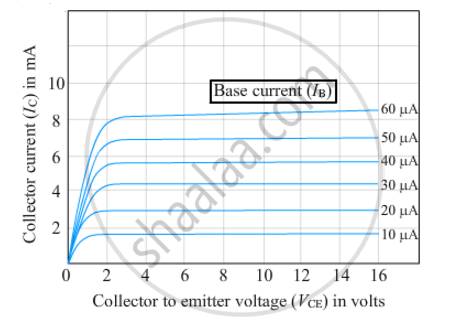# Define the Terms :Current Amplification Factor. How Are These Determined Using Typical Input and Output Characteristics? - Physics

Define the terms :Current amplification factor. How are these determined using typical input and output characteristics?

#### Solution

Current amplification factor (r) is defined as the ratio of change in emitter current (ΔIE) to the change in base current (ΔIB).

r=(DeltaI_E)/(DeltaI_B)Concept: Junction Transistor - Transistor: Structure and Action
Is there an error in this question or solution?